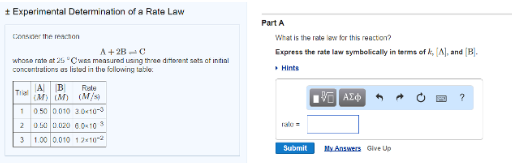# Problem: Consider the reaction A + 2B → Cwhose rate at 25°C was measured using three different sets of initial concentrations as listed in the following table: Part AWhat is the rate law for this reaction? Express the rate law symbolically in terms of k, [Al] and [B].

###### FREE Expert Solution
87% (106 ratings)###### Problem Details

Consider the reaction

A + 2B → C

whose rate at 25°C was measured using three different sets of initial concentrations as listed in the following table:

Part A

What is the rate law for this reaction? Express the rate law symbolically in terms of k, [Al] and [B].